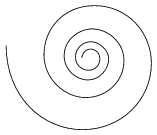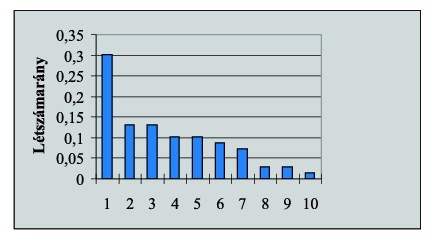Mathematical and Physical Journal
for High Schools
Issued by the MATFUND Foundation
 Already signed up? New to KöMaL?# Exercises and problems in InformaticsJanuary 2002

I. 13. Besides the usual decimal representation, integers can also be written in base -10 as follows:

x=x0+(-10).x1+(-10)2.x2+...+(-10)n.xn,

where 0$\displaystyle le$xi$\displaystyle le$9. In this base, every integer can be represented as a signless integer. Your program (I13.pas, I13.c, ...) should convert a number having at most 4 digits in base 10 into base -10, or vice versa, from base -10 to base 10.

Examples.

DecimalBase -10
33
-614(=-10+4)
34174(=100-70+4)
-7288(=-80+8)
163243(=200-40+3)
-5271533(=-1000+500-30+3)
152619686(=10000-9000+600-80+6)
199418014(=10000-8000+0-10+4)

(10 points)

I. 14. Archimedes' spiral keeps a constant distance between the corresponding arcs during consecutive revolutions. In the case of logarithmic spiral, however, this distance is multiplied by a constant in each revolution. Write a program (I14.pas, I14.c, ...) which reads the number of revolutions, then displays an Archimedes' spiral and a logarithmic one, as in the figure on page 46. (10 points)Archimedes' spiral Logarithmic spiral

I. 15. Individuals of an animal population have a maximal lifespan of 10 years, and we store the number of individuals of all 10 age groups. We also specify-for each age group-what the chance of dying of an individual in a year is (mortality rate), as well as the average number of offsprings of an individual ( birth rate).

Prepare a sheet (I15.xls) which contains the death and birth rates, the initial number of individuals, and then, upon pressing a key (or keys), it computes the number of individuals in each age group in the next year, and also creates a diagram showing the proportion of each age group relative to the whole population. Upon pressing another key (or keys), the program should restart from the initial time with the initial number of individuals. If the number of individuals became a real number during the calculations, it should be rounded.

Example. (Assuming that every age group contains 1000 individuals in the first time step.)

 Birth rate: Mortality rate: Time: 2 0,1 0,1 Age group 1: 2080 0,1 0,1 Age group 2: 900 0,4 0,3 Age group 3: 900 1,1 0,3 Age group 4: 700 0,9 0,4 Age group 5: 700 0,4 0,5 Age group 6: 600 0,3 0,8 Age group 7: 500 0,2 0,8 Age group 8: 200 0,1 0,9 Age group 9: 200 0 1 Age group 10: 100 Population: 6880(10 points)